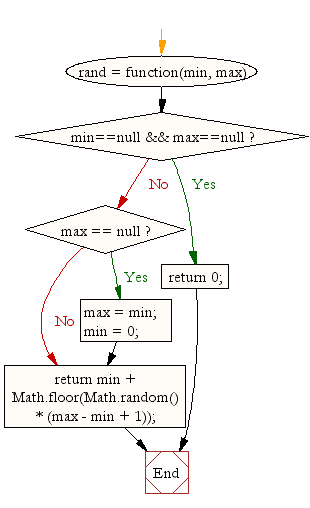# JavaScript: Generate a random integer

## JavaScript Math: Exercise-4 with Solution

Write a JavaScript function to generate a random integer.

Test Data :
console.log(rand(20,1));
console.log(rand(1,10));
console.log(rand(6));
console.log(rand());
15
5
1
0

Pictorial Presentation:Sample Solution:-

HTML Code:

``````<!DOCTYPE html>
<html>
<meta charset="utf-8">
<title>Javascript random function</title>
<body>

</body>
</html>
```
```

JavaScript Code:

``````rand = function(min, max) {
if (min==null && max==null)
return 0;

if (max == null) {
max = min;
min = 0;
}
return min + Math.floor(Math.random() * (max - min + 1));
};
console.log(rand(20,1));
console.log(rand(1,10));
console.log(rand(6));
console.log(rand());
```
```

Sample Output:

```4
1
2
0
```

Flowchart:Live Demo:

See the Pen javascript-math-exercise-4 by w3resource (@w3resource) on CodePen.

Improve this sample solution and post your code through Disqus

What is the difficulty level of this exercise?

Test your Programming skills with w3resource's quiz.

﻿